# Acids and BasesPage 1

#### PREVIEW

Slide 1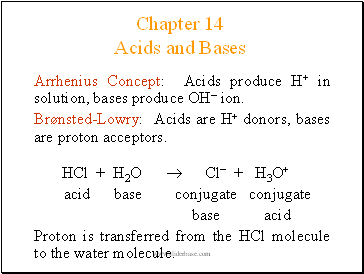## Acids and Bases

Chapter 14

Arrhenius Concept: Acids produce H+ in solution, bases produce OH ion.

Brønsted-Lowry: Acids are H+ donors, bases are proton acceptors.

HCl + H2O  Cl + H3O+

acid base conjugate conjugate

base acid

Proton is transferred from the HCl molecule to the water molecule.

Slide 2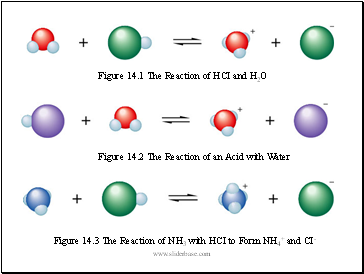Figure 14.1 The Reaction of HCI and H2O

Figure 14.2 The Reaction of an Acid with Water

Figure 14.3 The Reaction of NH3 with HCI to Form NH4+ and CI-

Slide 3## Conjugate Acid/Base Pairs

HA(aq) + H2O(l)  H3O+(aq) + A(aq)

conj conj conj conj

acid 1 base 2 acid 2 base 1

Conjugate base: everything that remains of the acid molecule after a proton is lost.

Conjugate acid: formed when the proton is transferred to the base.

A conjugate acid-base pair consists of two substances related to each other by the donating and accepting of a single proton.

Slide 4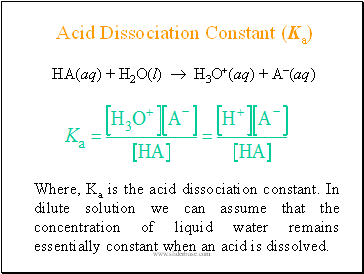## Acid Dissociation Constant (Ka)

HA(aq) + H2O(l)  H3O+(aq) + A(aq)

Where, Ka is the acid dissociation constant. In dilute solution we can assume that the concentration of liquid water remains essentially constant when an acid is dissolved.

Slide 5## Acid Strength

The strength of an acid is defined by the equilibrium position of its dissociation

(ionization) reaction:

HA(aq) + H2O(l) H3O+(aq) + A-(aq)

Strong Acid:

Its equilibrium position lies far to the right. (HNO3)

Yields a weak conjugate base. (NO3)

Common strong acids are H2SO4, HCl, HNO3, HClO4

Slide 6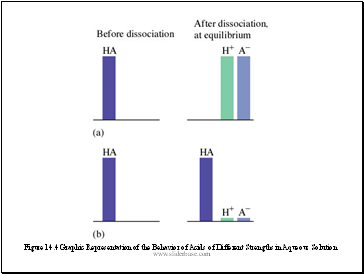Figure 14.4 Graphic Representation of the Behavior of Acids of Different Strengths in Aqueous Solution

Slide 7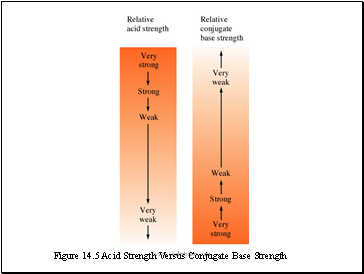Figure 14.5 Acid Strength Versus Conjugate Base Strength

Slide 8Acid Strength (continued)

Weak Acid:

Its equilibrium lies far to the left. (CH3COOH)

Yields a much stronger (it is relatively strong) conjugate base than water. (CH3COO)

Common weak acids are H3PO4, HNO2, HOCl, organic acids (-COOH).

Slide 9Figure 14.6 A Strong Acid (a) and a Weak Acid (b) in Water

Go to page:
1  2  3  4